Скачать презентацию Essential AP Microeconomics Formulas AVERAGE PRODUCT AP

50e6c7a907dd811dd14c03a850823225.ppt

• Количество слайдов: 49Essential AP Microeconomics FormulasAVERAGE PRODUCT (AP)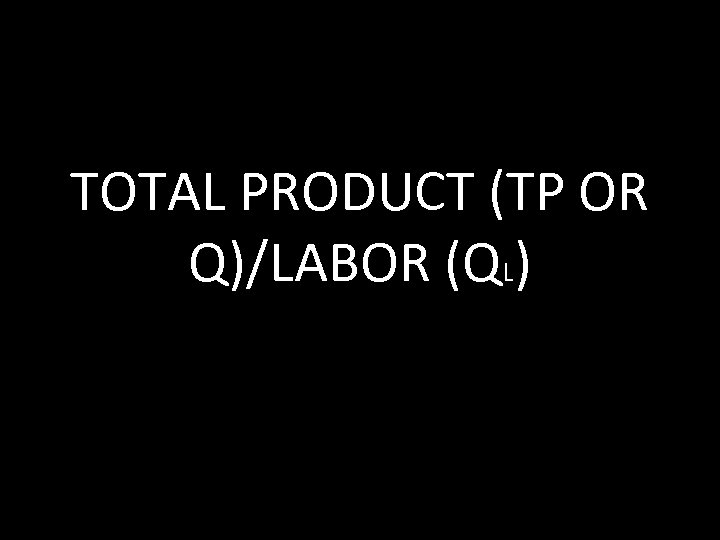TOTAL PRODUCT (TP OR Q)/LABOR (QL)MARGINAL PRODUCT (MP)ΔTP/ΔQLPROFIT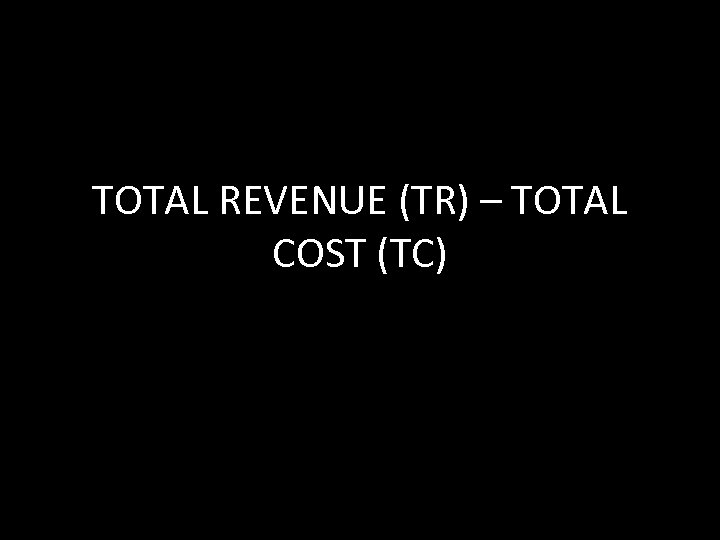TOTAL REVENUE (TR) – TOTAL COST (TC)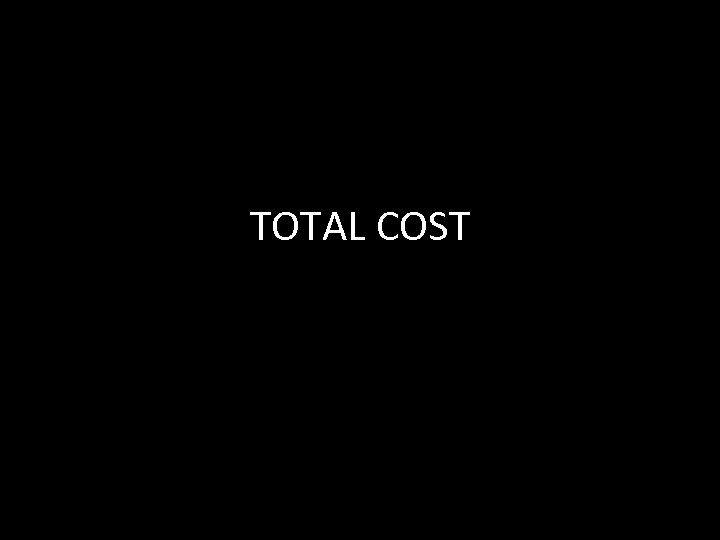TOTAL COST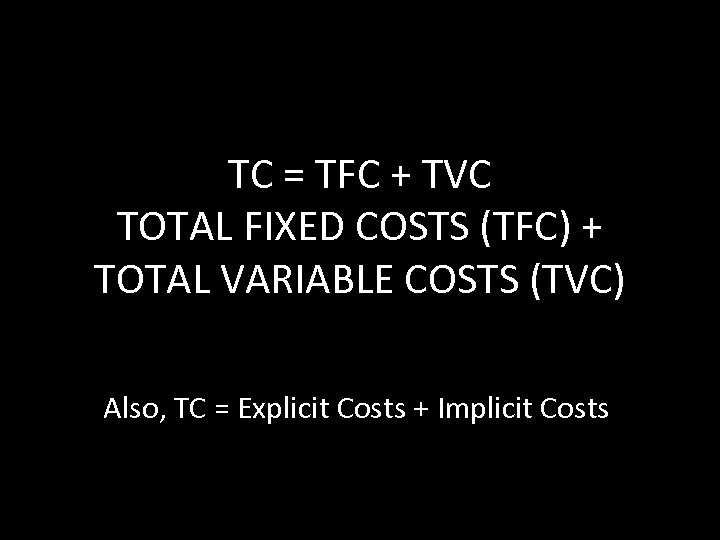TC = TFC + TVC TOTAL FIXED COSTS (TFC) + TOTAL VARIABLE COSTS (TVC) Also, TC = Explicit Costs + Implicit Costs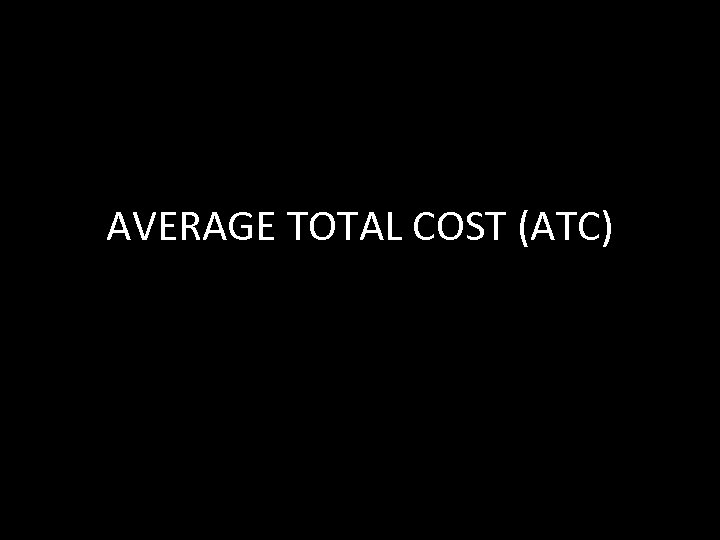AVERAGE TOTAL COST (ATC)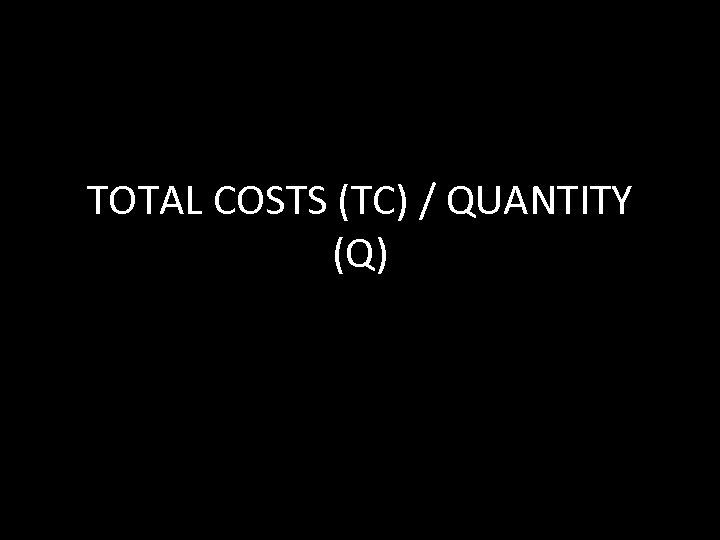TOTAL COSTS (TC) / QUANTITY (Q)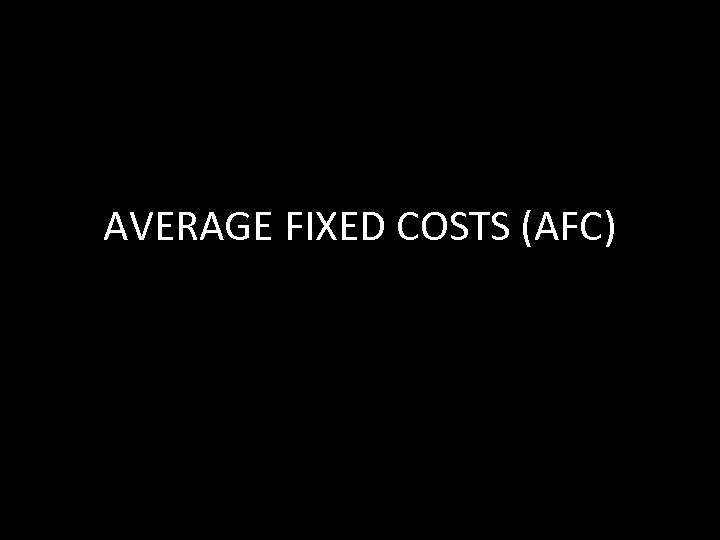AVERAGE FIXED COSTS (AFC)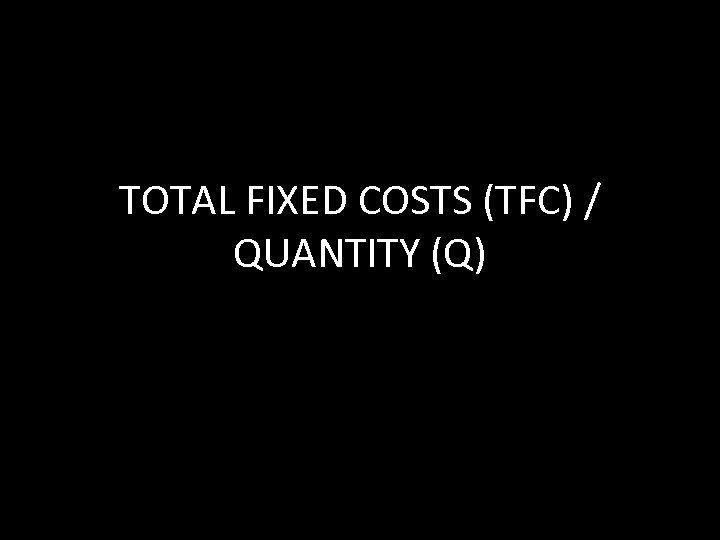TOTAL FIXED COSTS (TFC) / QUANTITY (Q)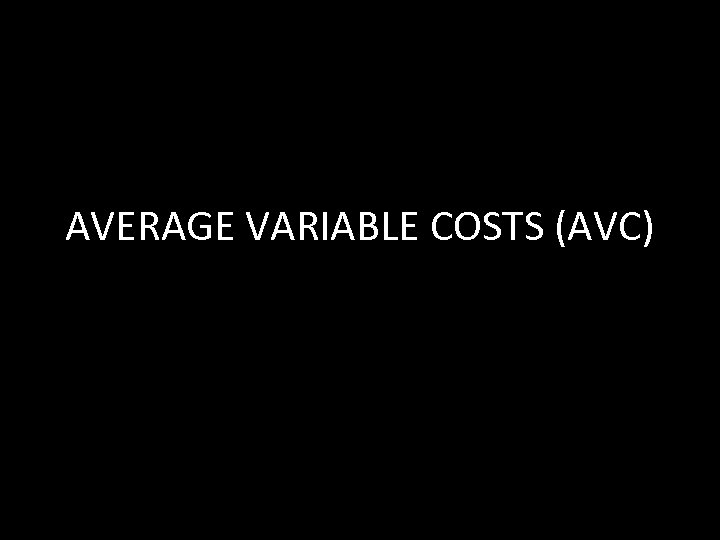AVERAGE VARIABLE COSTS (AVC)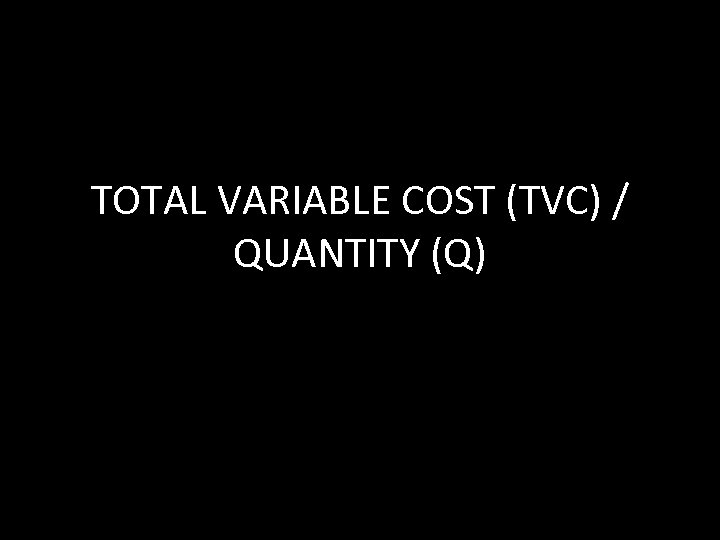TOTAL VARIABLE COST (TVC) / QUANTITY (Q)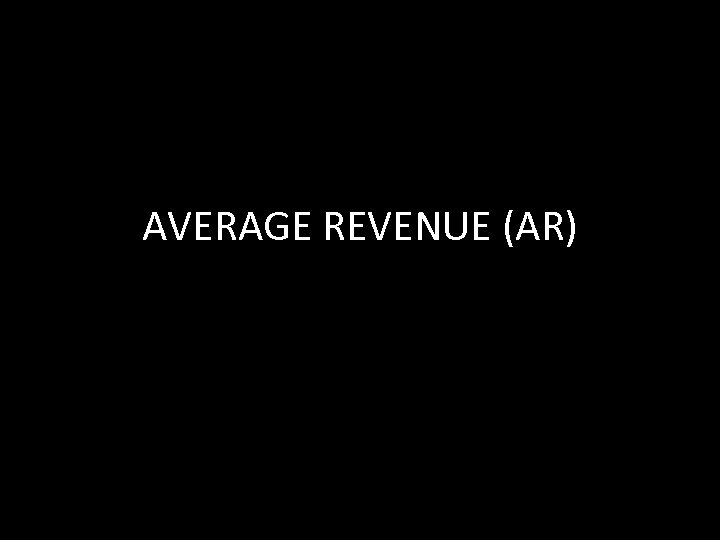AVERAGE REVENUE (AR)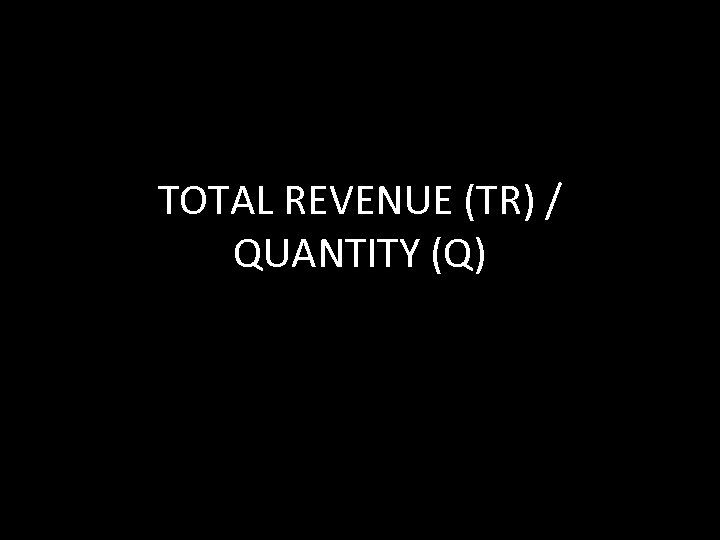TOTAL REVENUE (TR) / QUANTITY (Q)IN PERFECT COMPETITION…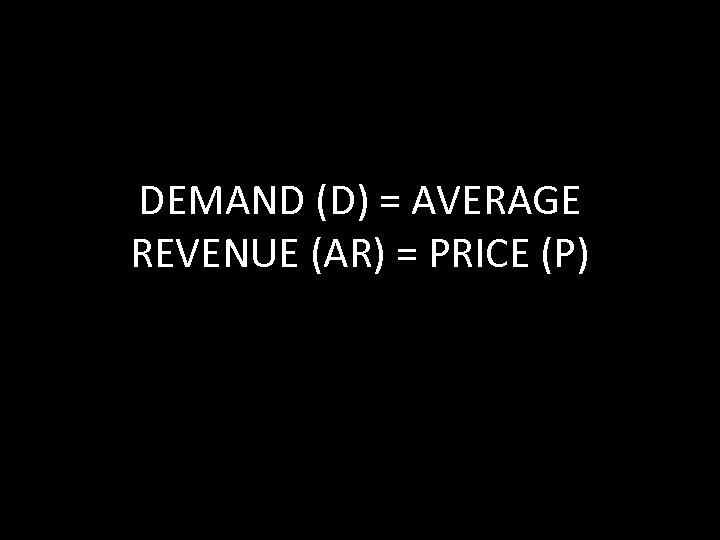DEMAND (D) = AVERAGE REVENUE (AR) = PRICE (P)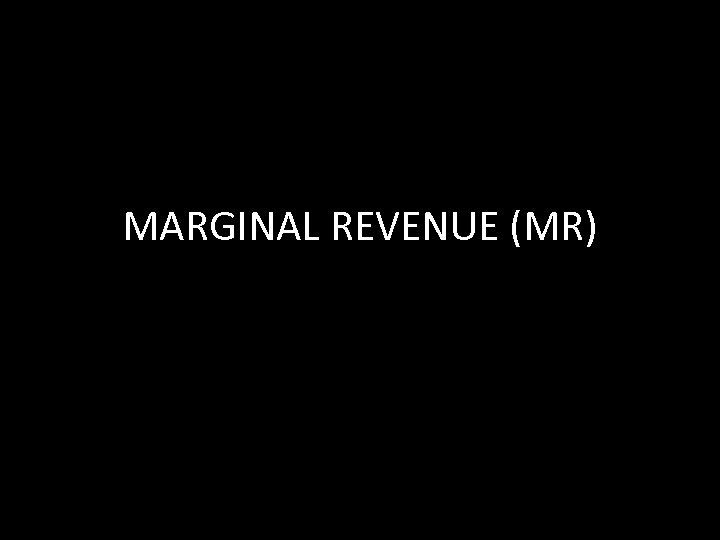MARGINAL REVENUE (MR)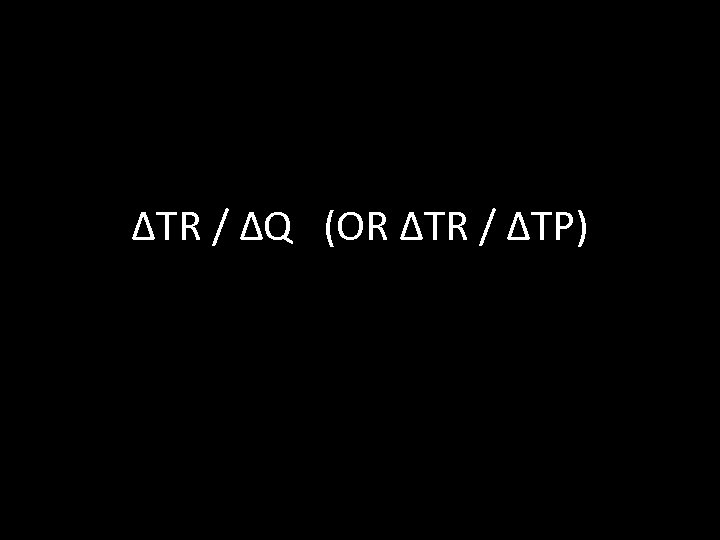ΔTR / ΔQ (OR ΔTR / ΔTP)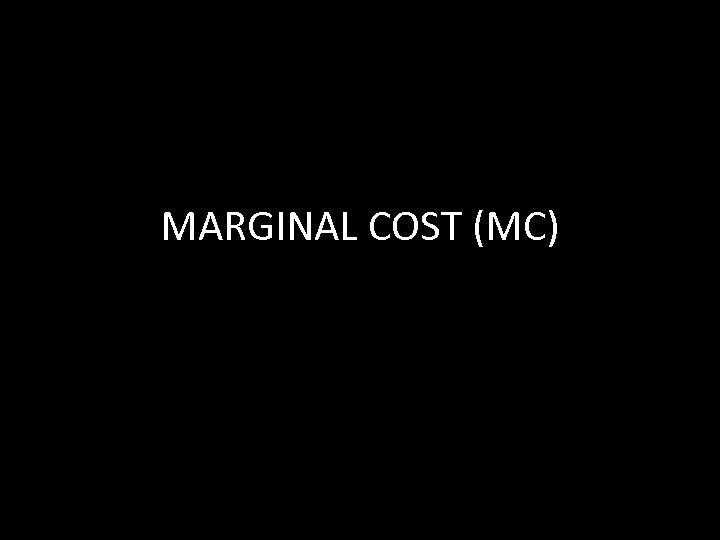MARGINAL COST (MC)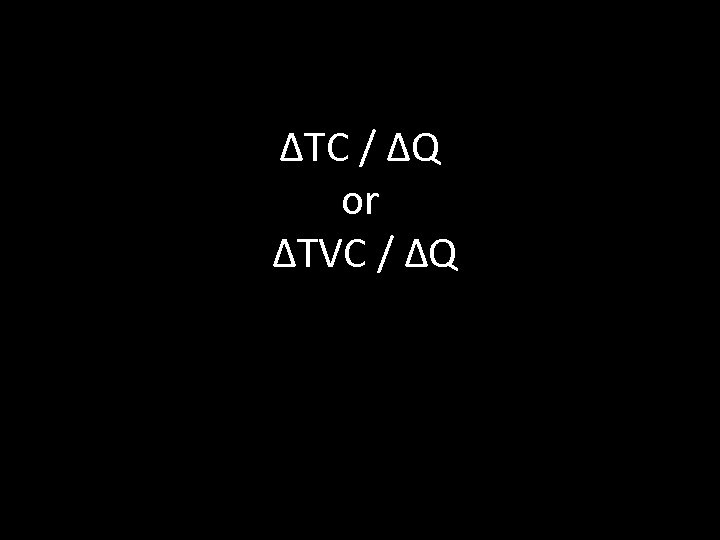ΔTC / ΔQ or ΔTVC / ΔQPROFIT MAXIMIZATION POINTWHERE MC = MR“BREAKEVEN” POINT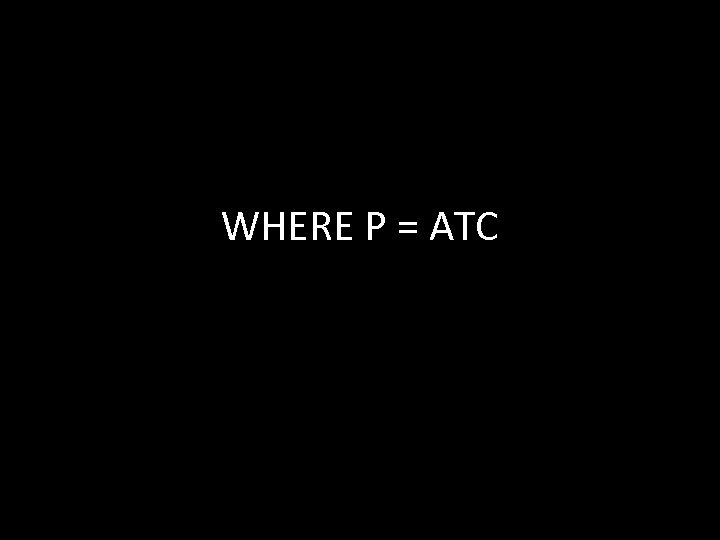WHERE P = ATCSHUTDOWN POINTWHERE P = AVCUtility Maximization occurs when…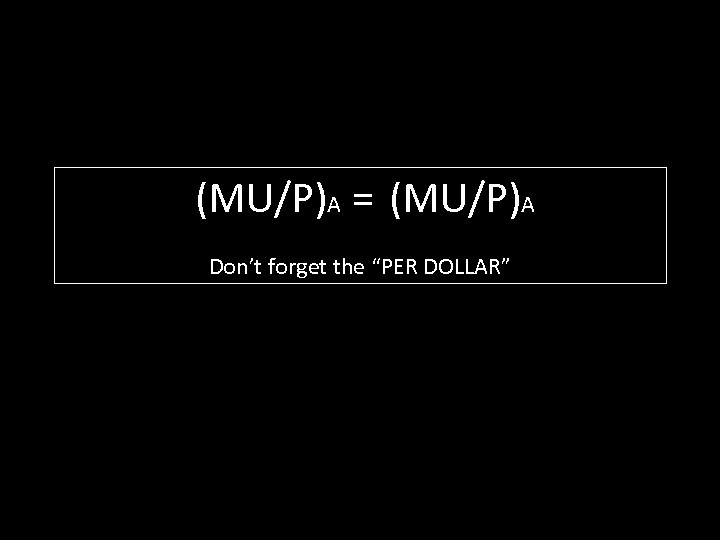(MU/P)A = (MU/P)A Don’t forget the “PER DOLLAR”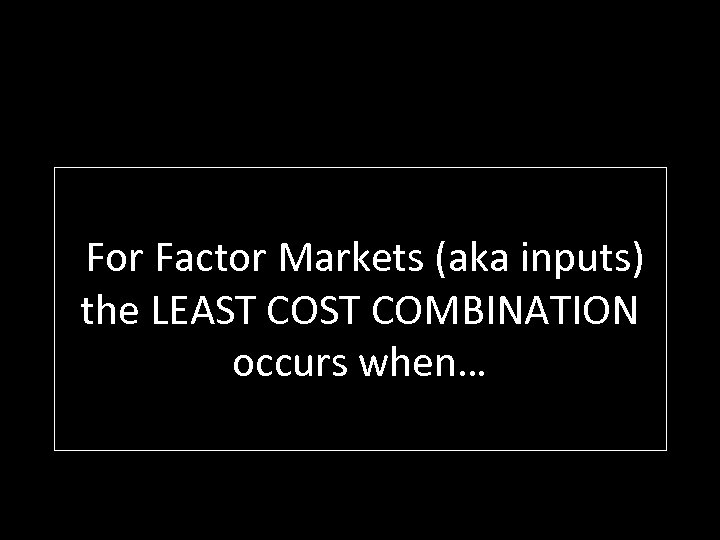For Factor Markets (aka inputs) the LEAST COMBINATION occurs when…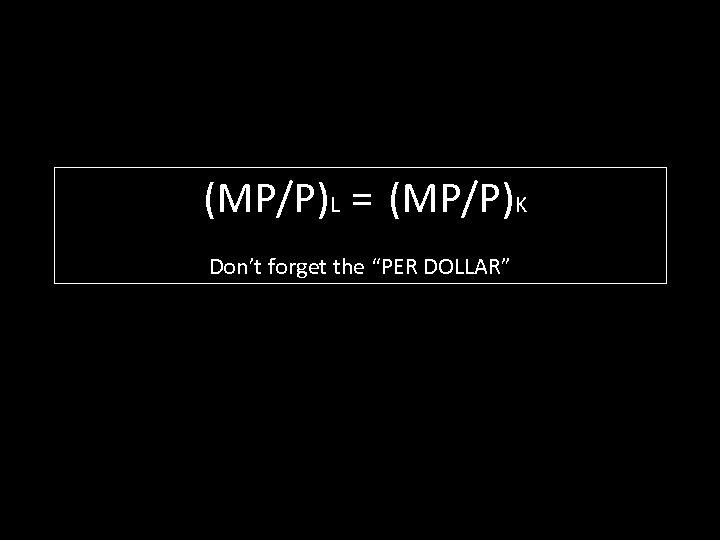(MP/P)L = (MP/P)K Don’t forget the “PER DOLLAR”What is true of MR and TR when Ed is INELASTIC?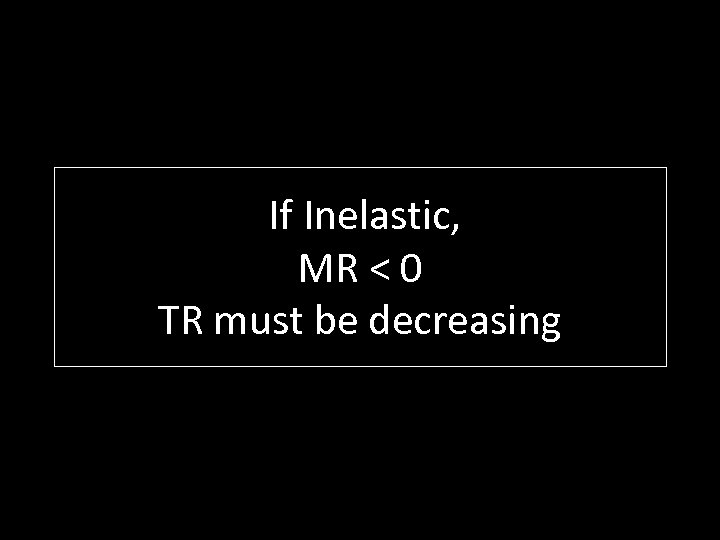If Inelastic, MR < 0 TR must be decreasingWhat is true of MR and TR when Ed is ELASTIC?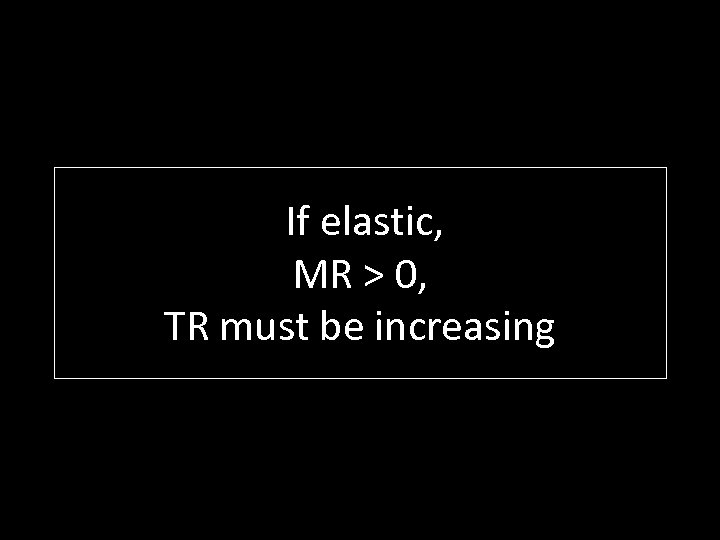If elastic, MR > 0, TR must be increasingFor Factor Markets, what determines a firm’s profitmaximizing hiring decision?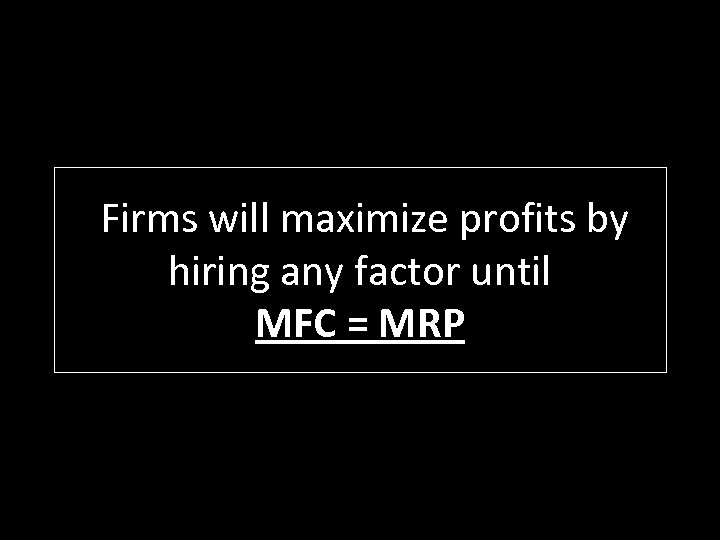Firms will maximize profits by hiring any factor until MFC = MRP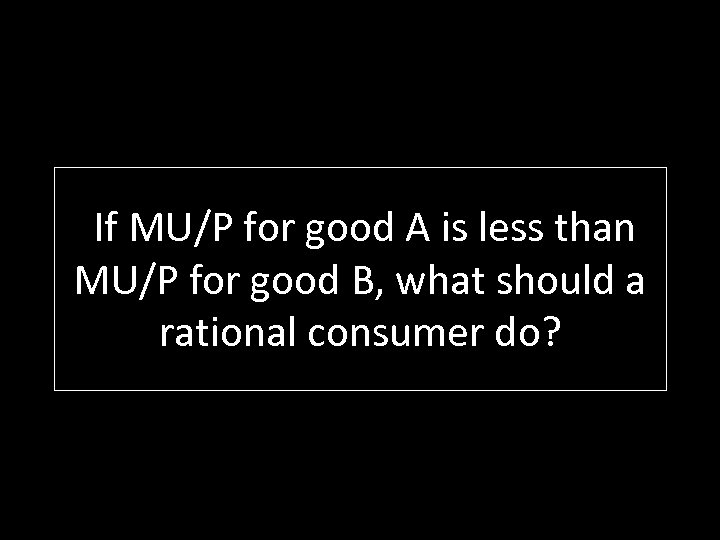If MU/P for good A is less than MU/P for good B, what should a rational consumer do?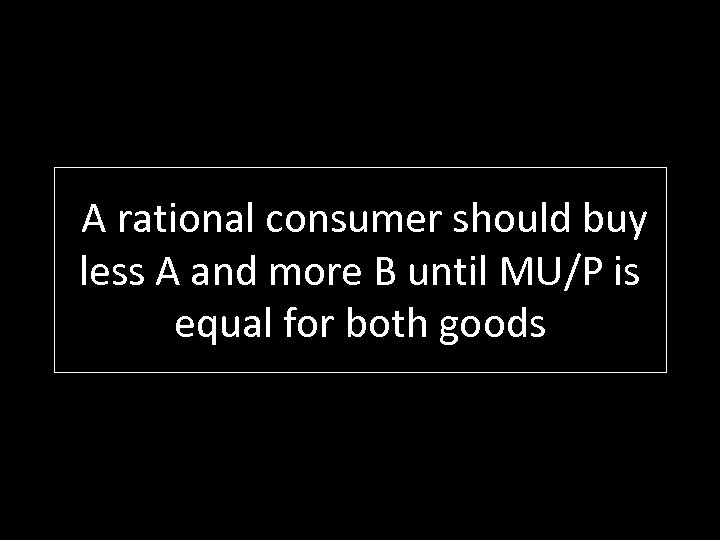A rational consumer should buy less A and more B until MU/P is equal for both goodsIf Marginal Private Benefit (MPB) is less than Marginal Social Benefit (MSB), what is likely the reason? How could this be ‘fixed’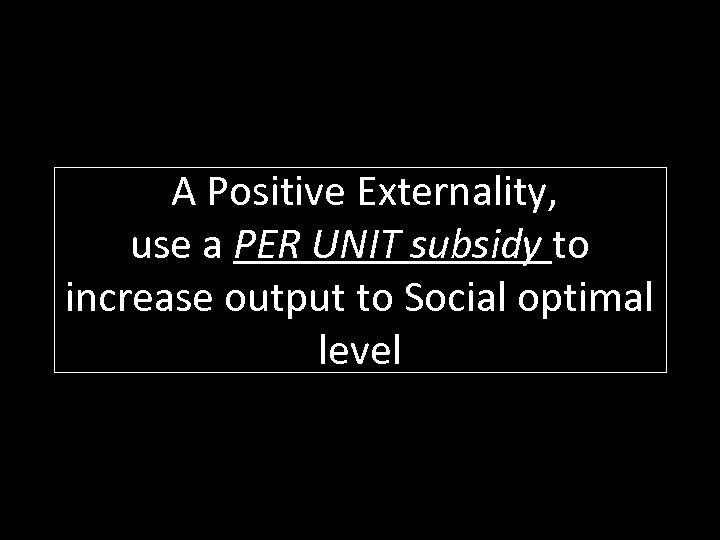A Positive Externality, use a PER UNIT subsidy to increase output to Social optimal level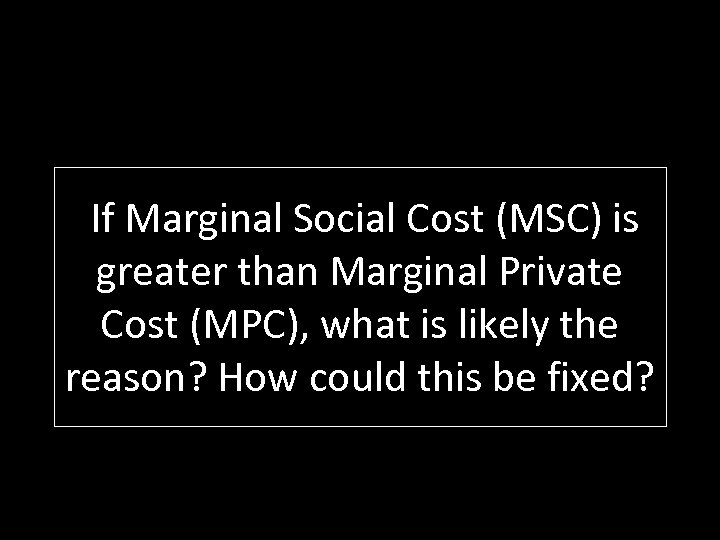If Marginal Social Cost (MSC) is greater than Marginal Private Cost (MPC), what is likely the reason? How could this be fixed?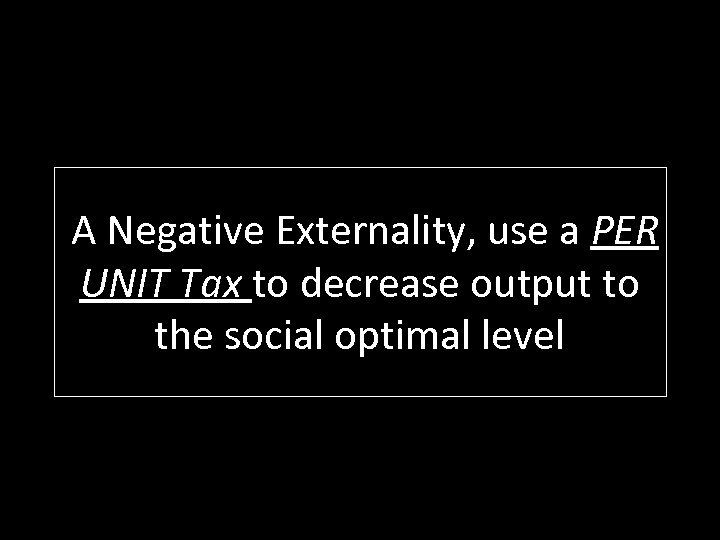A Negative Externality, use a PER UNIT Tax to decrease output to the social optimal level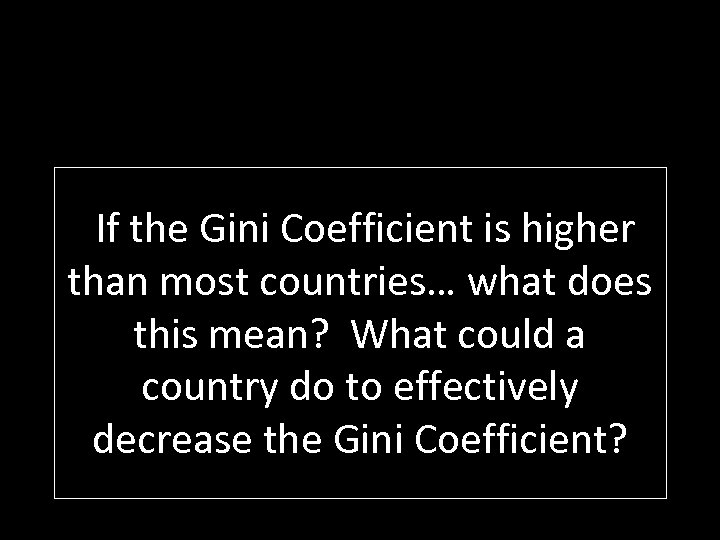If the Gini Coefficient is higher than most countries… what does this mean? What could a country do to effectively decrease the Gini Coefficient?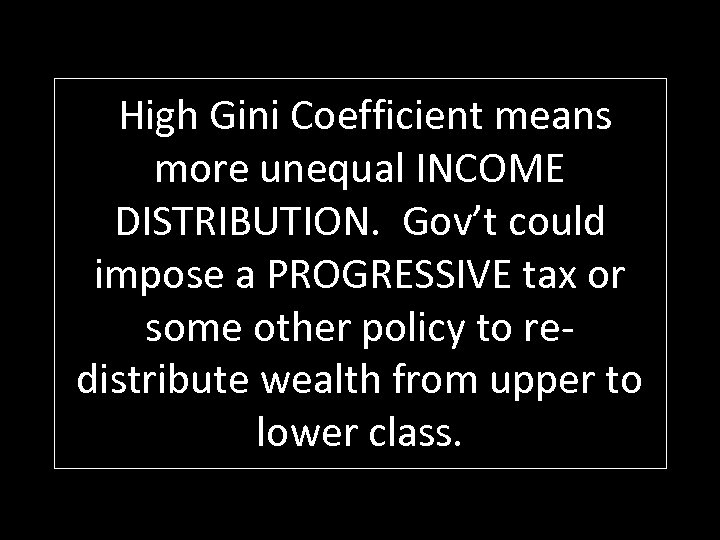High Gini Coefficient means more unequal INCOME DISTRIBUTION. Gov’t could impose a PROGRESSIVE tax or some other policy to redistribute wealth from upper to lower class.What graph will always be both ALLOCATIVELY and PRODUCTIVELY efficient?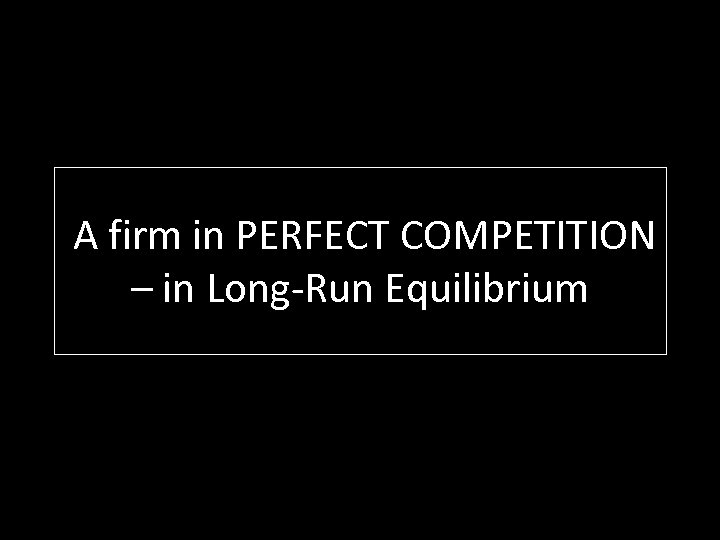A firm in PERFECT COMPETITION – in Long-Run Equilibrium